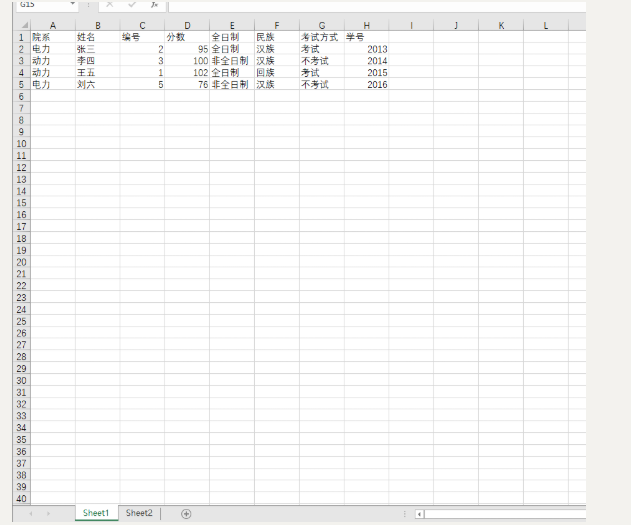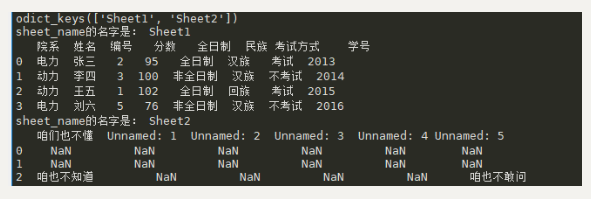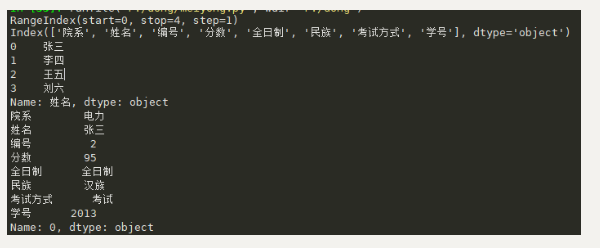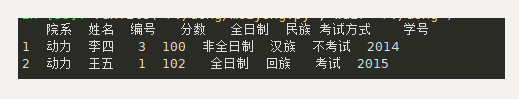出租广告位,需要合作请联系站长

+关注

## python对excel操作获取某一列，某一行的值，对某一列信息筛选

python对excel操作大全详解（获取某一列，某一行的值，对某一列信息筛选）

## 使用pd读取有多个sheet的excel

`pandas`读取的常用格式`pd.read_excel(file, sheet_name)`，其中`sheetname`可以使用数字进行替代，从0开始，默认为0

`pandas`写入的格式为`data.to_excel('filename',sheet_name='A')```````import pandas as pd
path = 'G:\动力系\新建文件夹\什么.xls'
print(data.keys())#查看sheet的名字
for sh_name in data.keys():
print('sheet_name的名字是：',sh_name)
print(sh_data)

``````## 获得某一行，某一列的值

pd对excel的相关基本操作看下面的链接

https://blog.csdn.net/weixin_43245453/article/details/90056884

``````import pandas as pd
path = 'G:\动力系\新建文件夹\什么.xls'
print(data.index)#获取行的索引名称
print(data.columns)#获取列的索引名称
print(data['姓名'])#获取列名为姓名这一列的内容
print(data.loc)#获取行名为0这一行的内容
``````## 对某一列的信息进行筛选

``````import pandas as pd
path = 'G:\动力系\新建文件夹\什么.xls'
result = data.loc[data['院系'] == '动力']#获取列明为院系，内容为动力的内容
print(result)
``````0 0

pdf(new) 更多>